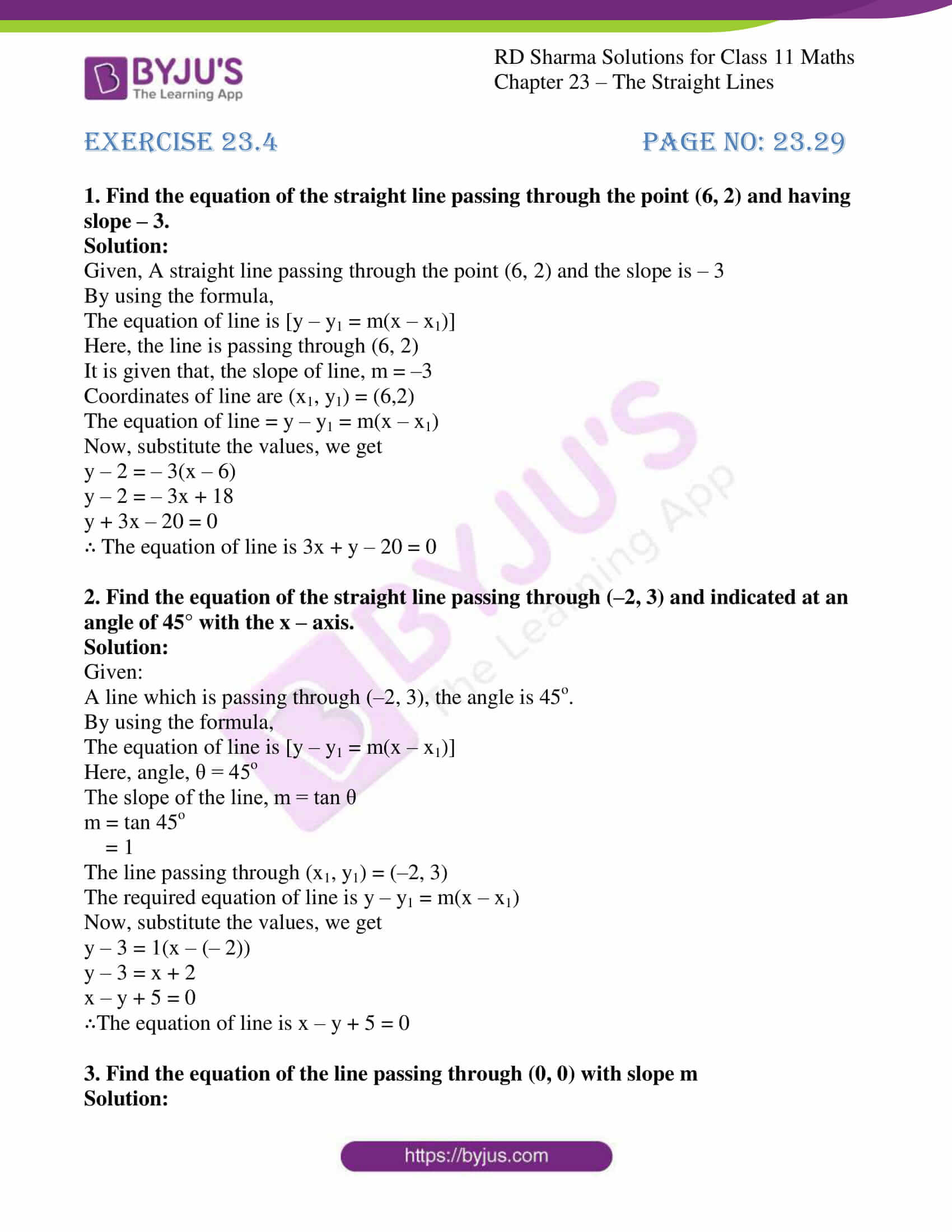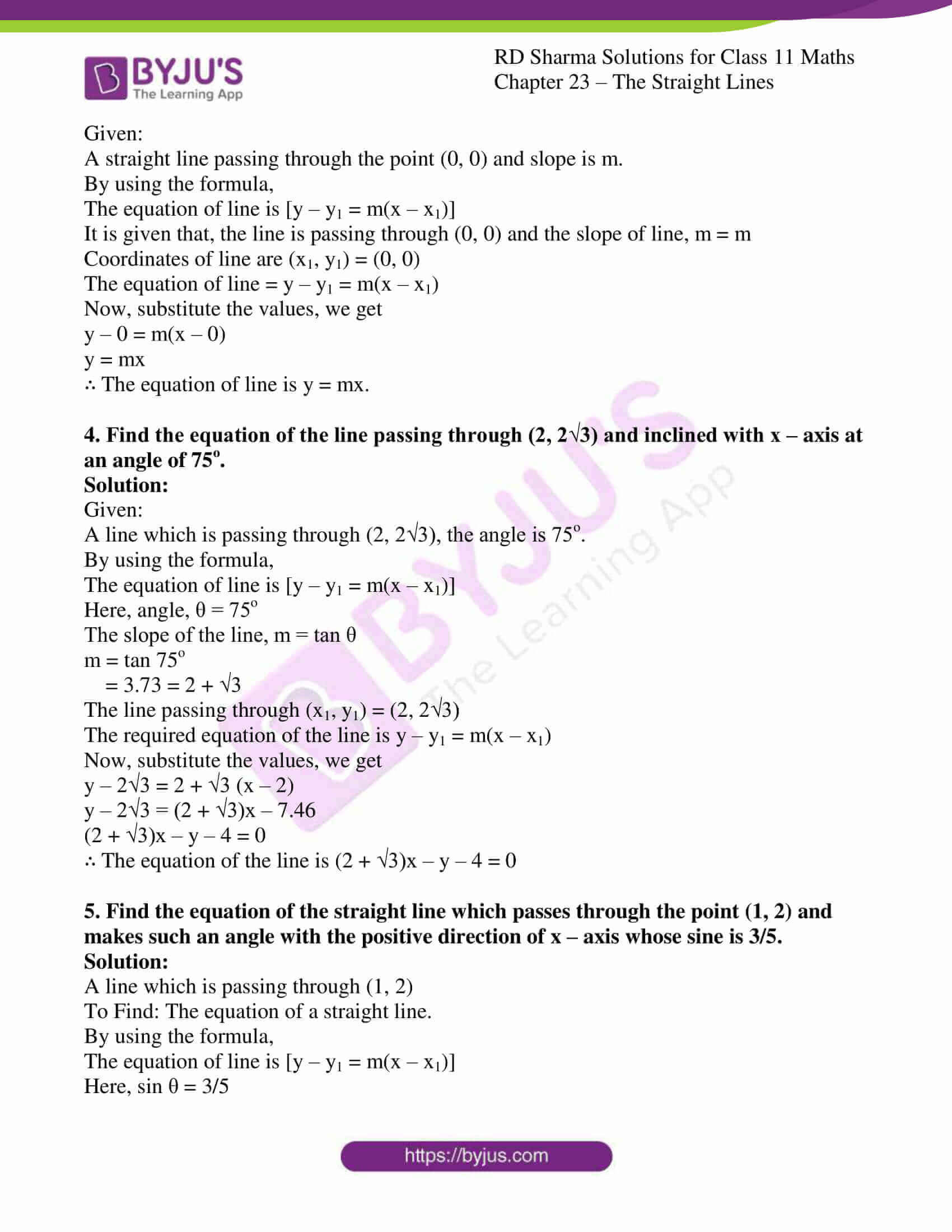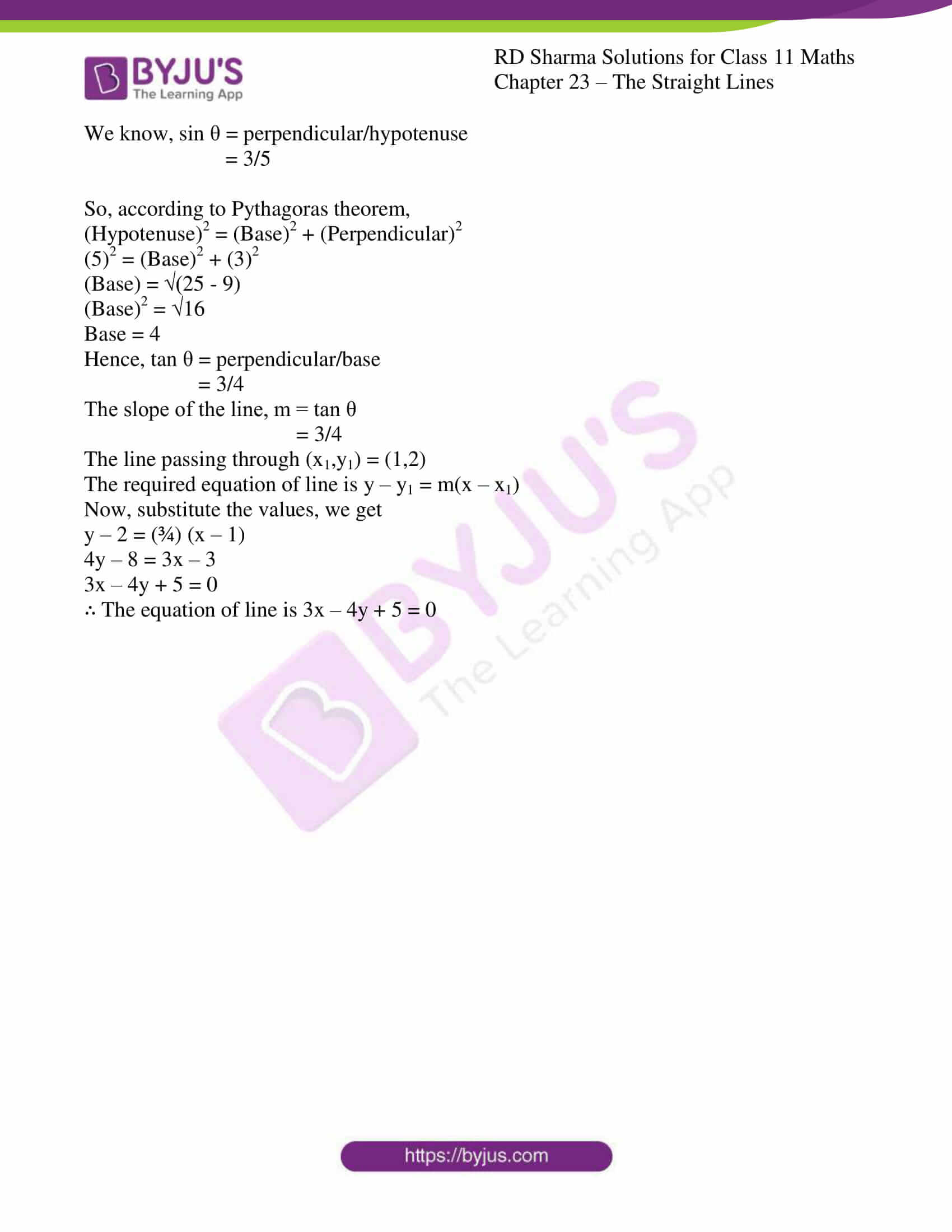# RD Sharma Solutions for Class 11 Chapter 23 - The Straight Lines Exercise 23.4

Here, in this exercise we shall discuss one of the different forms of the equation of a straight line i.e., point-slope form of a line with illustrations, for better understanding of the concepts. The problems from the textbook are solved by a set of teachers at BYJU’S who have vast experience in the education industry. Experts, keeping in mind the understanding abilities of students, design the solutions. With the aim to help students speed up their exam preparation, the solutions are made readily available in pdf format. In order to perform well in the annual exam, download the RD Sharma Class 11 Maths Solutions pdf from the links provided below.

## Download the pdf of RD Sharma Solutions for Class 11 Maths Exercise 23.4 Chapter 23 – The Straight Lines### Also, access other exercises of RD Sharma Solutions for Class 11 Maths Chapter 23 – The Straight Lines

Exercise 23.1 Solutions

Exercise 23.2 Solutions

Exercise 23.3 Solutions

Exercise 23.5 Solutions

Exercise 23.6 Solutions

Exercise 23.7 Solutions

Exercise 23.8 Solutions

Exercise 23.9 Solutions

Exercise 23.10 Solutions

Exercise 23.11 Solutions

Exercise 23.12 Solutions

Exercise 23.13 Solutions

Exercise 23.14 Solutions

Exercise 23.15 Solutions

Exercise 23.16 Solutions

Exercise 23.17 Solutions

Exercise 23.18 Solutions

Exercise 23.19 Solutions

### Access answers to RD Sharma Solutions for Class 11 Maths Exercise 23.4 Chapter 23 – The Straight Lines

#### EXERCISE 23.4 PAGE NO: 23.29

1. Find the equation of the straight line passing through the point (6, 2) and having slope – 3.

Solution:

Given, A straight line passing through the point (6, 2) and the slope is – 3

By using the formula,

The equation of line is [y – y1 = m(x – x1)]

Here, the line is passing through (6, 2)

It is given that, the slope of line, m = –3

Coordinates of line are (x1, y1) = (6,2)

The equation of line = y – y1 = m(x – x1)

Now, substitute the values, we get

y – 2 = – 3(x – 6)

y – 2 = – 3x + 18

y + 3x – 20 = 0

∴ The equation of line is 3x + y – 20 = 0

2. Find the equation of the straight line passing through (–2, 3) and indicated at an angle of 45° with the x – axis.

Solution:

Given:

A line which is passing through (–2, 3), the angle is 45o.

By using the formula,

The equation of line is [y – y1 = m(x – x1)]

Here, angle, θ = 45o

The slope of the line, m = tan θ

m = tan 45o

= 1

The line passing through (x1, y1) = (–2, 3)

The required equation of line is y – y1 = m(x – x1)

Now, substitute the values, we get

y – 3 = 1(x – (– 2))

y – 3 = x + 2

x – y + 5 = 0

∴The equation of line is x – y + 5 = 0

3. Find the equation of the line passing through (0, 0) with slope m

Solution:

Given:

A straight line passing through the point (0, 0) and slope is m.

By using the formula,

The equation of line is [y – y1 = m(x – x1)]

It is given that, the line is passing through (0, 0) and the slope of line, m = m

Coordinates of line are (x1, y1) = (0, 0)

The equation of line = y – y1 = m(x – x1)

Now, substitute the values, we get

y – 0 = m(x – 0)

y = mx

∴ The equation of line is y = mx.

4. Find the equation of the line passing through (2, 2√3) and inclined with x – axis at an angle of 75o.

Solution:

Given:

A line which is passing through (2, 2√3), the angle is 75o.

By using the formula,

The equation of line is [y – y1 = m(x – x1)]

Here, angle, θ = 75o

The slope of the line, m = tan θ

m = tan 75o

= 3.73 = 2 + √3

The line passing through (x1, y1) = (2, 2√3)

The required equation of the line is y – y1 = m(x – x1)

Now, substitute the values, we get

y – 2√3 = 2 + √3 (x – 2)

y – 2√3 = (2 + √3)x – 7.46

(2 + √3)x – y – 4 = 0

∴ The equation of the line is (2 + √3)x – y – 4 = 0

5. Find the equation of the straight line which passes through the point (1, 2) and makes such an angle with the positive direction of x – axis whose sine is 3/5.

Solution:

A line which is passing through (1, 2)

To Find: The equation of a straight line.

By using the formula,

The equation of line is [y – y1 = m(x – x1)]

Here, sin θ = 3/5

We know, sin θ = perpendicular/hypotenuse

= 3/5

So, according to Pythagoras theorem,

(Hypotenuse)2 = (Base)2 + (Perpendicular)2

(5)2 = (Base)2 + (3)2

(Base) = √(25 – 9)

(Base)2 = √16

Base = 4

Hence, tan θ = perpendicular/base

= 3/4

The slope of the line, m = tan θ

= 3/4

The line passing through (x1,y1) = (1,2)

The required equation of line is y – y1 = m(x – x1)

Now, substitute the values, we get

y – 2 = (¾) (x – 1)

4y – 8 = 3x – 3

3x – 4y + 5 = 0

∴ The equation of line is 3x – 4y + 5 = 0